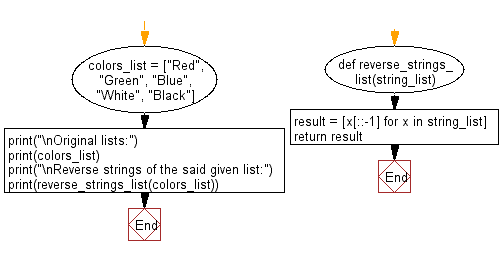﻿ Python: Reverse strings in a given list of string values - w3resource# Python: Reverse strings in a given list of string values

## Python List: Exercise - 123 with Solution

Write a Python program to reverse strings in a given list of string values.

Sample Solution:

Python Code:

``````def reverse_strings_list(string_list):
result = [x[::-1] for x in string_list]
return result

colors_list = ["Red", "Green", "Blue", "White", "Black"]
print("\nOriginal lists:")
print(colors_list)
print("\nReverse strings of the said given list:")
print(reverse_strings_list(colors_list))
```
```

Sample Output:

```Original lists:
['Red', 'Green', 'Blue', 'White', 'Black']

Reverse strings of the said given list:
['deR', 'neerG', 'eulB', 'etihW', 'kcalB']
```

Flowchart:## Visualize Python code execution:

The following tool visualize what the computer is doing step-by-step as it executes the said program:

Python Code Editor:

Have another way to solve this solution? Contribute your code (and comments) through Disqus.

What is the difficulty level of this exercise?

Test your Python skills with w3resource's quiz

﻿

## Python: Tips of the Day

Floor Division:

When we speak of division we normally mean (/) float division operator, this will give a precise result in float format with decimals.

For a rounded integer result there is (//) floor division operator in Python. Floor division will only give integer results that are round numbers.

```print(1000 // 300)
print(1000 / 300)```

Output:

```3
3.3333333333333335```